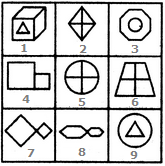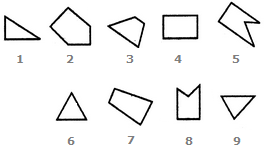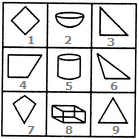# Non Verbal Reasoning - Grouping of Images

Exercise : Grouping of Images - Section 1
Directions to Solve

In each of the following questions, group the given figures into three classes using each figure only once.

1.

Group the given figures into three classes using each figure only once.1,4 ; 2,3 ; 5,6
1,5 ; 2,6 ; 4,3
1,6 ; 2,3 ; 4,5
1,2 ; 3,6 ; 4,5
Explanation:

(1, 4), (2, 3) and (5, 6) are three different pairs of identical figures.

2.

Group the given figures into three classes using each figure only once.1,3,9 ; 2,5,6 ; 4,7,8
1,3,9 ; 2,7,8 ; 4,5,6
1,2,4 ; 3,5,7 ; 6,8,9
1,3,6 ; 2,4,8 ; 5,7,9
Explanation:

1, 3, 9 have one element placed inside a different element.

2, 5, 6 contain two mutually perpendicular lines dividing the figure into four parts.

4, 7, 8 have two similar elements (unequal in size) attached to each other.

3.

Group the given figures into three classes using each figure only once.7,8,9 ; 2,4,3 ; 1,5,6
1,3,2 ; 4,5,7 ; 6,8,9
1,6,8 ; 3,4,7 ; 2,5,9
1,6,9 ; 3,4,7 ; 2,5,8
Explanation:

1, 6, 9, are all triangles.

3, 4, 7 are all four-sided figures.

2, 5, 8 are all five-sided figures.

4.

Group the given figures into three classes using each figure only once.1,2,3 ; 4,5,6 ; 7,8,9
1,3,5 ; 2,4,6 ; 7,8,9
1,5,9 ; 3,6,2 ; 4,7,8
1,9,7 ; 2,8,5 ; 3,4,6
Explanation:

1, 2, 3 are figures composed of two straight lines.

4, 5, 6 are figures composed of three straight lines.

7, 8, 9 are figures composed of four straight lines.

5.

Group the given figures into three classes using each figure only once.1,4,7 ; 2,5,8 ; 3,6,9
1,4,7 ; 2,5,9 ; 3,6,7
1,3,4 ; 2,5,8 ; 6,7,9
1,2,3 ; 4,5,6 ; 7,8,9